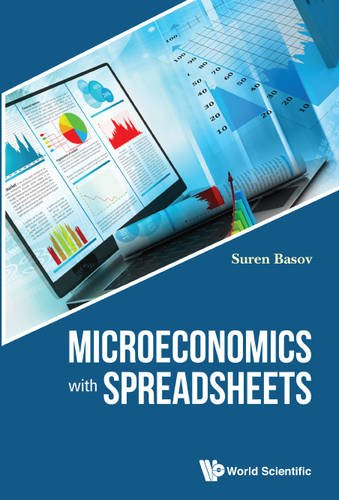Description

Microeconomics studies the choices made by individuals under conditions of scarcity of resources and time and the interaction between different decision makers. Scarcity forces economic actors to choose one opportunity among many. Microeconomics with Spreadsheets starts with the mathematical preliminaries and covers consumer theory, producer theory, general equilibrium, game theory, market structure and economics of information.

The reader will use numerical tools to analyse problems that cannot be analysed analytically. There is a natural synergy between rigorous proofs and numerical methods, since before using a numerical method one should also prove that a solution exists and analyse whether it is unique, and therefore be able to interpret properly the output of a program.

Part I Mathematical Preliminaries
Chapter 1 Constraint Optimization

Part II Market Interactions
Chapter 2 The Consumer Theory
Chapter 3 The Producer Theory 39
Chapter 4 General Equilibrium 51
Chapter 5 Choice and Uncertainty

Part III Strategic Interactions
Chapter 6 Game Theory
Chapter 7 Theory of Imperfect Competition and Industry Structure

Part IV The Economics of Information
Chapter 8 Hidden Information Models
Chapter 9 Hidden Action
Chapter 10 Introduction to Auctions

Part V Mathematical Appendix
Chapter 11 The Formal Logic
Chapter 12 Basics of Set Theory
Chapter 13 Solutions of Some Equations Systems of Simultaneous Equations
Chapter 14 Basics of Calculus